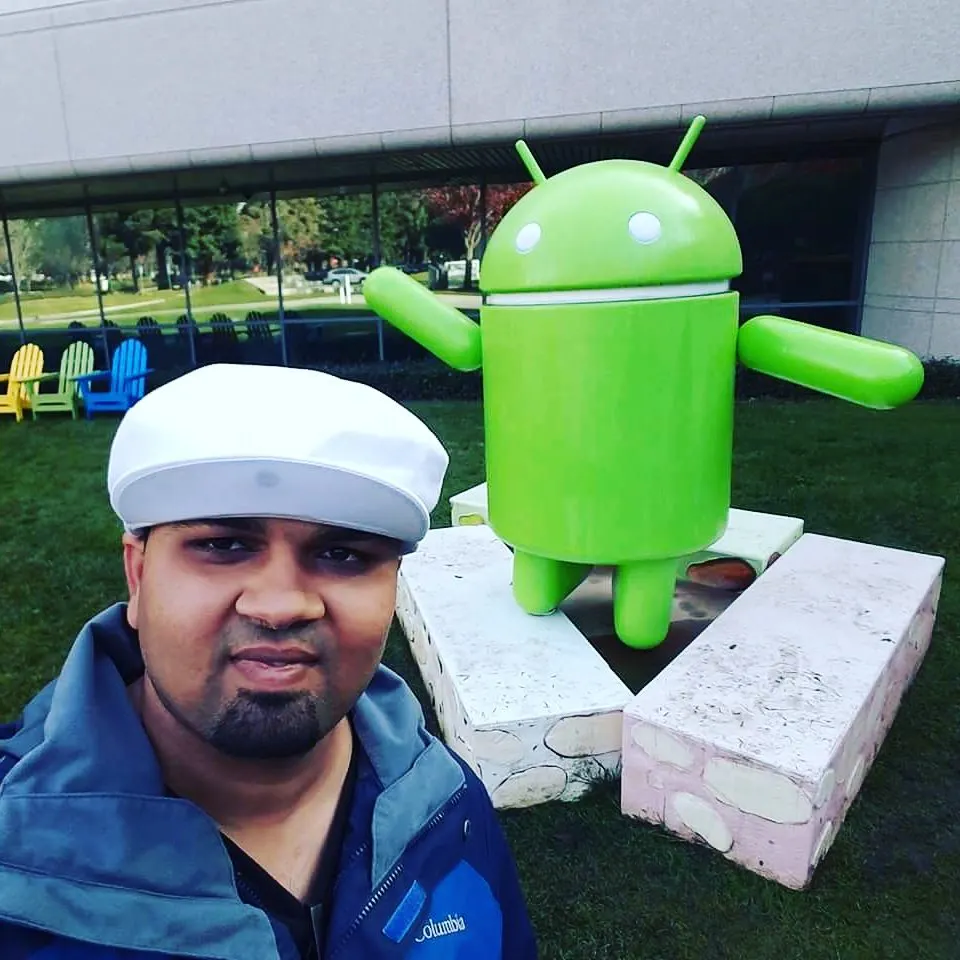# PHPoC - Arduino Self Balancing Robot with BT+Web Control

Arduino Self Balancing Robot using PHPoC, Gyroscope and Acceleraometer

IntermediateWork in progressOver 1 day10,134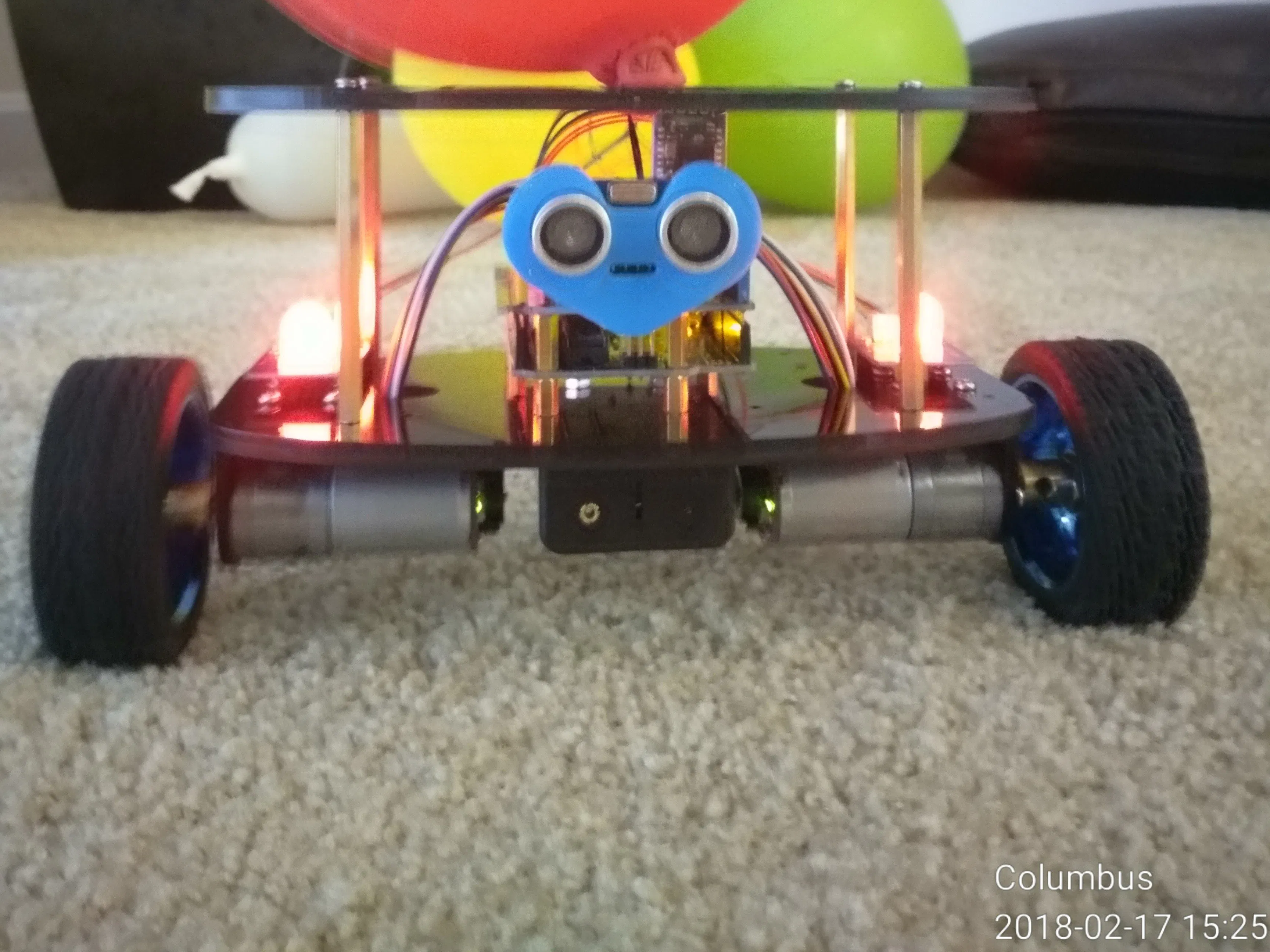## Things used in this project

### Hardware componentsArduino UNO
×1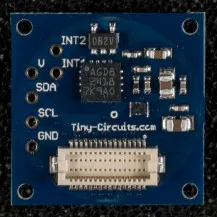TinyCircuits TinyShield Gyroscope
×1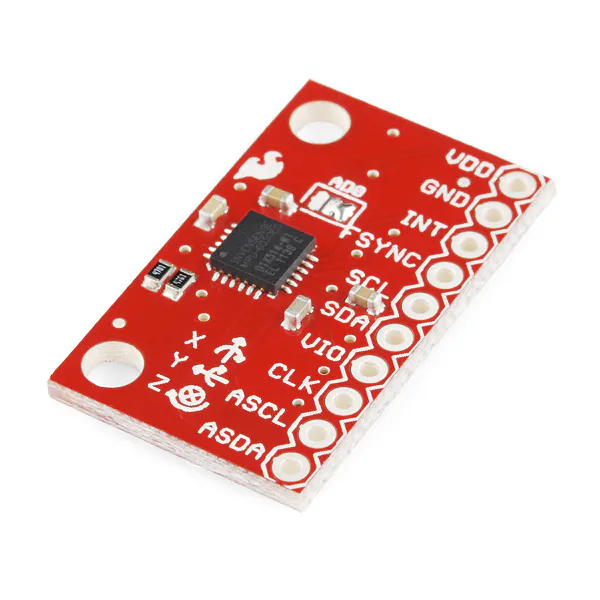SparkFun Triple Axis Accelerometer and Gyro Breakout - MPU-6050
×1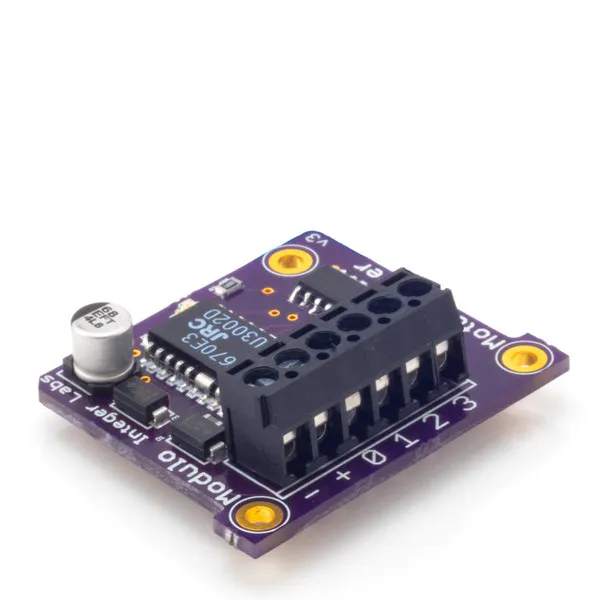Modulo Motor Driver
×1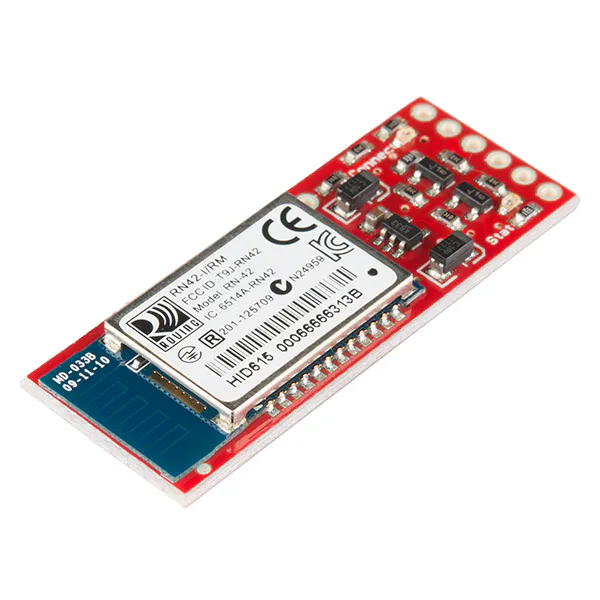SparkFun Bluetooth Modem - BlueSMiRF Silver
×1Ultrasonic Sensor - HC-SR04 (Generic)
×1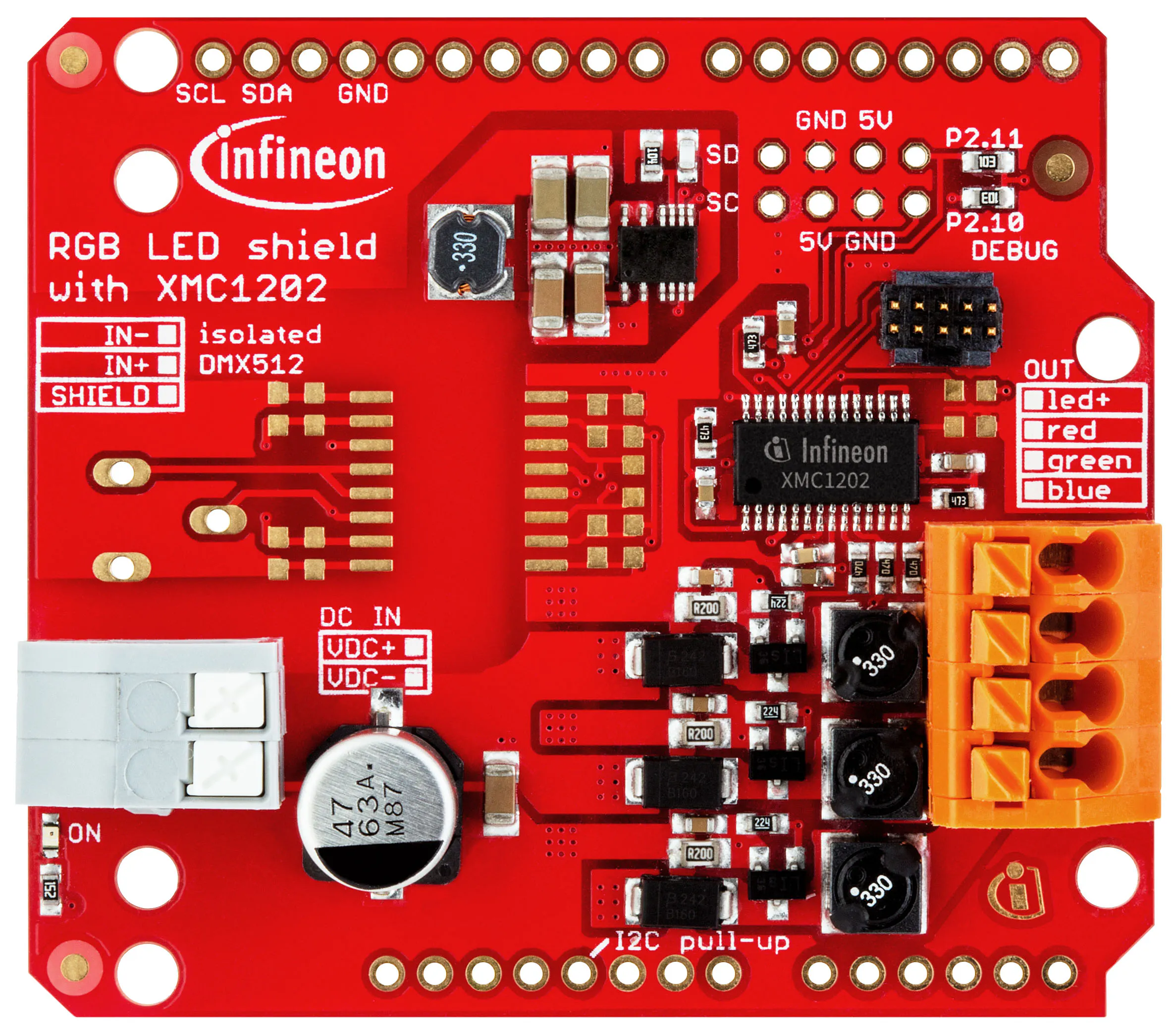Infineon RGB LED Lighting Shield with XMC1202
×1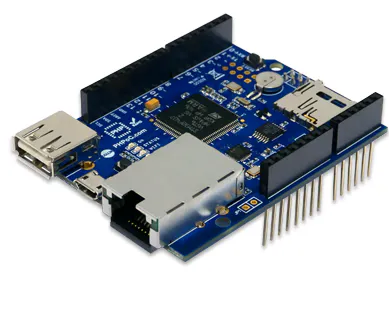PHPoC Shield for Arduino
×1

## Schematics

### Schematics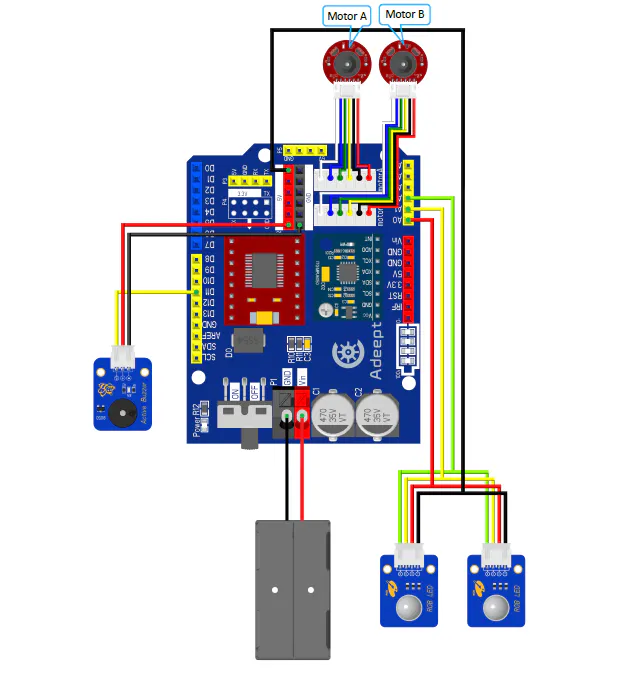## Code

### Arduino UNO 3

C/C++
```#include "PinChangeInt.h"
#include "MsTimer2.h"
#include "I2Cdev.h"
#include "MPU6050_6Axis_MotionApps20.h"
#include "Wire.h"

MPU6050 mpu; //Instantiate an MPU6050 object with the object name mpu
Adeept_Balance2WD balancecar;//Instantiate an balance object with the object name balancecar
Adeept_KalmanFilter kalmanfilter;//Instantiate an KalmanFilter object with the object name kalmanfilter
Adeept_Distance Dist;//Instantiate an distance object with the object name Dist

//mode = 0:Remote control via Bluetooth mode
//mode = 1:Obstacle avoidance by ultrasonic mode
//mode = 2:Following  mode
int mode = 0;
int motorRun = 0;//0:Stop;  1:Go ahead;  2;Backwards;  3:Turn left;  4:Turn right;

int16_t ax, ay, az, gx, gy, gz;
//TB6612FNG Drive module control signal
#define IN1M 7
#define IN2M 6
#define IN3M 13
#define IN4M 12
#define PWMA 9
#define PWMB 10
#define STBY 8
//Speed PID control is realized by using speed code counting
#define PinA_left 2  //Interrupt 0
#define PinA_right 4 //Interrupt 1

const int RPin = A0; //RGB LED Pin R
const int GPin = A1; //RGB LED Pin G
const int BPin = A2; //RGB LED Pin B

/****************************Declare a custom variable*****************/
int time;
byte inByte; //The serial port receives the byte
int num;
double Setpoint;                                         //Angle DIP setpoint, input, and output
double Setpoints, Outputs = 0;                           //Speed DIP setpoint, input, and output
double kp = 30, ki = 0.1, kd = 0.58;//kp = 38, ki = 0, kd = 0.58;//Need you to modify the parameters kp = 30, ki = 0.1, kd = 0.58;
double kp_speed = 3.6, ki_speed = 0.1058, kd_speed = 0.0; // Need you to modify the parameters kp_speed = 3.6, ki_speed = 0.1058, kd_speed = 0.0;
double kp_turn = 28, ki_turn = 0, kd_turn = 0.29;        //Rotate PID setting
//Steering PID parameters
double setp0 = 0, dpwm = 0, dl = 0; //Angle balance point, PWM difference, dead zone, PWM1, PWM2
float value;

/********************angle data*********************/
float Q;
float Angle_ax; //The angle of inclination calculated from the acceleration
float Angle_ay;
float K1 = 0.05; // The weight of the accelerometer
float angle0 = 0.00; //Mechanical balance angle
int slong;

/***************Kalman_Filter*********************/
float Q_angle = 0.001, Q_gyro = 0.005; //Angle data confidence, angular velocity data confidence
float R_angle = 0.5 , C_0 = 1;
float timeChange = 5; //Filter method sampling time interval milliseconds
float dt = timeChange * 0.001; //Note: The value of dt is the filter sampling time

/******************* speed count ************/
volatile long count_right = 0;//Use the volatile long type to ensure that the value is valid for external interrupt pulse count values used in other functions
volatile long count_left = 0;//Use the volatile long type to ensure that the value is valid for external interrupt pulse count values used in other functions
int speedcc = 0;

/*******************************Pulse calculation*****************************/
int lz = 0;
int rz = 0;
int rpluse = 0;
int lpluse = 0;

/********************Turn the parameters of rotation**********************/
int turncount = 0; //
float turnoutput = 0;

/****************Bluetooth control volume*******************/
int front = 0;//Forward variable
int back = 0;//Backward variables
int turnl = 0;//Turn left mark
int turnr = 0;//Turn right
int spinl = 0;//Left rotation mark
int spinr = 0;//Right turn mark

/***************Ultrasonic velocity******************/
int distance;
int detTime=0;

const int buzzerPin = 11;  // define pin for buzzer

/*Pulse calculation*/
void countpluse(){
lz = count_left;
rz = count_right;

count_left = 0;
count_right = 0;

lpluse = lz;
rpluse = rz;

if ((balancecar.pwm1 < 0) && (balancecar.pwm2 < 0)){//Car movement direction to determine: back when (PWM is the motor voltage is negative) pulse number is negative
rpluse = -rpluse;
lpluse = -lpluse;
}else if ((balancecar.pwm1 > 0) && (balancecar.pwm2 > 0)){//Car movement direction to determine: forward (PWM is the motor voltage is positive) pulse number is negative
rpluse = rpluse;
lpluse = lpluse;
}else if ((balancecar.pwm1 < 0) && (balancecar.pwm2 > 0)){////Car movement direction to determine: right rotation, the right pulse number is positive, the number of left pulse is negative.
rpluse = rpluse;
lpluse = -lpluse;
}else if ((balancecar.pwm1 > 0) && (balancecar.pwm2 < 0)){//Car movement direction to determine: left rotation, the right pulse number is negative, the number of left pulse is positive.
rpluse = -rpluse;
lpluse = lpluse;
}
//To judge
balancecar.stopr += rpluse;
balancecar.stopl += lpluse;
//Every 5ms into the interruption, the number of pulses superimposed
balancecar.pulseright += rpluse;
balancecar.pulseleft += lpluse;
}
/*Angle PD*/
void angleout(){
balancecar.angleoutput = kp * (kalmanfilter.angle + angle0) + kd * kalmanfilter.Gyro_x;//PD angle loop control
}
/*Interrupt timing 5ms timer interrupt*/
void inter(){
sei();
countpluse();                                     //Pulse superposition of sub - functions
mpu.getMotion6(&ax, &ay, &az, &gx, &gy, &gz);     //IIC gets MPU6050 six axis data ax ay az gx gy gz
kalmanfilter.angleTest(ax, ay, az, gx, gy, gz, dt, Q_angle, Q_gyro,R_angle,C_0,K1);  //Get angle and Kaman filter
angleout();                                       //Angle loop PD control
speedcc++;
if (speedcc >= 8){                                //50ms into the speed loop control
Outputs = balancecar.speedPiOut(kp_speed,ki_speed,kd_speed,front,back,setp0);
speedcc = 0;
}
turncount++;
if (turncount > 2){                                //10ms into the rotation control
turnoutput = balancecar.turnSpin(turnl,turnr,spinl,spinr,kp_turn,kd_turn,kalmanfilter.Gyro_z);  //Rotary subfunction
turncount = 0;
}
balancecar.posture++;
balancecar.pwma(Outputs,turnoutput,kalmanfilter.angle,kalmanfilter.angle6,turnl,turnr,spinl,spinr,front,back,kalmanfilter.accelz,IN1M,IN2M,IN3M,IN4M,PWMA,PWMB);//car total PWM output
if(mode!=0&&detTime>=100){
distance = Dist.getDistanceCentimeter();
}
detTime++;
if(detTime>100){detTime=0;}
}

void setup() {
// TB6612FNGN drive module control signal initialization
pinMode(IN1M, OUTPUT);//Control the direction of the motor 1, 01 for the forward rotation, 10 for the reverse
pinMode(IN2M, OUTPUT);
pinMode(IN3M, OUTPUT);//Control the direction of the motor 2, 01 for the forward rotation, 10 for the reverse
pinMode(IN4M, OUTPUT);
pinMode(PWMA, OUTPUT);//Left motor PWM
pinMode(PWMB, OUTPUT);//Right motor PWM
pinMode(STBY, OUTPUT);//TB6612FNG enabled

//Initialize the motor drive module
digitalWrite(IN1M, 0);
digitalWrite(IN2M, 1);
digitalWrite(IN3M, 1);
digitalWrite(IN4M, 0);
digitalWrite(STBY, 1);
analogWrite(PWMA, 0);
analogWrite(PWMB, 0);

pinMode(PinA_left, INPUT);  //Speed code A input
pinMode(PinA_right, INPUT); //Speed code B input

pinMode(RPin, OUTPUT);   // set RPin to output mode
pinMode(GPin, OUTPUT);   // set GPin to output mode
pinMode(BPin, OUTPUT);   // set BPin to output mode

Dist.begin(5,3);//begin(int echoPin, int trigPin)
//Initialize the I2C bus
Wire.begin();
//Turn on the serial port and set the baud rate to 9600
//Communicate with the Bluetooth module
Serial.begin(9600);
delay(150);
//Initialize the MPU6050
mpu.initialize();
delay(2);
//5ms timer interrupt setting. Use timer2. Note: Using timer2 will affect the PWM output of pin3 and pin11.
//Because the PWM is used to control the duty cycle timer, so when using the timer should pay attention to
//see the corresponding timer pin port.
MsTimer2::set(5, inter);
MsTimer2::start();
}

void loop() {
//The main function of the cycle of detection and superposition of pulse, the determination of car speed.
//Use the level change both into the pulse superposition, increase the number of motor pulses to ensure
//the accuracy of the car.
attachInterrupt(0, Code_left, CHANGE);
attachPinChangeInterrupt(PinA_right, Code_right, CHANGE);
//Bluetooth control
case 'a': motorRun = 3;break;//go ahead
case 'b': motorRun = 1;break;//turn right
case 'c': motorRun = 2;break;//turn left
case 'd': motorRun = 4;break;//backwards
case 'e': mode = 0; motorRun = 0;break;
case 'f': mode = 1; break;
case 'g': mode = 2; break;
case 'h': digitalWrite(buzzerPin, HIGH);break;
case 'i': digitalWrite(buzzerPin, LOW); break;
}
}

if(mode==0){//Remote control via Bluetooth mode
switch(motorRun){
case 0: front = 0; back = 0; turnl = 0; turnr = 0; spinl = 0; spinr = 0; turnoutput = 0;  // control steering and reversing smart car
digitalWrite(RPin, LOW);digitalWrite(GPin, HIGH);digitalWrite(BPin, HIGH);//red led
break;
case 1: turnr = 1; // control smart 2WD balance turn right
digitalWrite(RPin, HIGH);digitalWrite(GPin, LOW); digitalWrite(BPin, HIGH);
break;
case 2: turnl = 1;// control smart 2WD balance turn left
digitalWrite(RPin, HIGH);digitalWrite(GPin, LOW); digitalWrite(BPin, HIGH);
break;
case 3: back = 50;// control 2WD balance car backwards
digitalWrite(RPin, HIGH);digitalWrite(GPin, LOW); digitalWrite(BPin, HIGH);
break;
case 4: front = -50;// control 2WD balance car go ahead
digitalWrite(RPin, HIGH);digitalWrite(GPin, LOW); digitalWrite(BPin, HIGH);
break;
default:break;
}
}
if(mode==1){//Obstacle avoidance by ultrasonic mode
if(distance<30){
front = -50;// control 2WD balance car go ahead
back = 0; turnl = 0; turnr = 0; spinl = 0; spinr = 0; turnoutput = 0;
digitalWrite(RPin, HIGH);digitalWrite(GPin, HIGH); digitalWrite(BPin, LOW);
}else if(distance<60&&distance>30){
turnl = 1; // control smart 2WD balance turn left
front = 0; back = 0; turnr = 0; spinl = 0; spinr = 0; turnoutput = 0;
digitalWrite(RPin, HIGH);digitalWrite(GPin, LOW); digitalWrite(BPin, LOW);
}else{
back = 50;// control 2WD balance car backwards
front = 0;  turnl = 0; turnr = 0; spinl = 0; spinr = 0; turnoutput = 0;
digitalWrite(RPin, HIGH);digitalWrite(GPin, LOW); digitalWrite(BPin, HIGH);
}
}
if(mode==2){//Following  mode
if(distance>=30&&distance<50){
back = 50;// control 2WD balance car backwards
digitalWrite(RPin, HIGH);digitalWrite(GPin, LOW); digitalWrite(BPin, HIGH);
}
if(distance<20&&distance>5){
front = -50;// control 2WD balance car go ahead
digitalWrite(RPin, HIGH);digitalWrite(GPin, LOW); digitalWrite(BPin, HIGH);
}else{
front = 0; back = 0; turnl = 0; turnr = 0; spinl = 0; spinr = 0; turnoutput = 0;  // control 2WD balance car stop
digitalWrite(RPin, LOW);digitalWrite(GPin, HIGH); digitalWrite(BPin, HIGH);
}
}
}
/*Left speed chart*/
void Code_left() {
count_left ++;
}
/*Right speed chart count*/
void Code_right() {
count_right ++;
}
```

### PHPoC

PHP
```<?php
include_once "config.php";
include_once "/lib/sc_envu.php";
if(!(\$wsm_width = envu_find(\$envu, "wsm_width")))
\$wsm_width = "400";
if(!(\$wsm_height = envu_find(\$envu, "wsm_height")))
\$wsm_height = "400";
if(!(\$baud = envu_find(\$envu, "wsm_baud")))
\$baud = "9600";
?>
<html>
<title>PHPoC Shield - Web Car Control for Arduino</title>
<meta name="viewport" content="width=device-width, initial-scale=0.7, maximum-scale=0.7">
<style>
.button {
background-color: #4CAF50; /* Green */
border: none;
color: white;
text-align: center;
text-decoration: none;
display: inline-block;
font-size: 16px;
margin: 4px 2px;
cursor: pointer;
}

.button2 {background-color: #008CBA;} /* Blue */
.button3 {background-color: #f44336;} /* Red */
.button4 {background-color: #e7e7e7; color: black;} /* Gray */
.button5 {background-color: #555555;} /* Black */
</style>

<script type="text/javascript">

var ws;
function init()
{
connect_onclick();
}
function connect_onclick()
{
if(ws == null)
{
var debug = document.getElementById("debug");

if((navigator.platform.indexOf("Win") != -1) && (ws_host_addr.charAt(0) == "["))
{
// network resource identifier to UNC path name conversion
}

debug.innerHTML = "<br>" + navigator.platform + " " + ws_host_addr;
ws = new WebSocket("ws://" + ws_host_addr + "/car_control", "text.phpoc");

document.getElementById("ws_state").innerHTML = "CONNECTING";

ws.onopen = ws_onopen;
ws.onclose = ws_onclose;
ws.onmessage = ws_onmessage;
}
else
ws.close();
}
function ws_onopen()
{
document.getElementById("ws_state").innerHTML = "<font color='blue'>CONNECTED</font>";
}

function ws_onclose()
{
document.getElementById("ws_state").innerHTML = "<font color='gray'>CLOSED</font>";

ws.onopen = null;
ws.onclose = null;
ws.onmessage = null;
ws = null;
}
function ws_onmessage(e_msg)
{
e_msg = e_msg || window.event; // MessageEvent

}

function sendCommand(btn)
{
{
if(btn.id=="whistle")
ws.send("A");

if(btn.id=="left")
ws.send("B");

if(btn.id=="right")
ws.send("C");

if(btn.id=="stop")
ws.send("D");
}
}

</script>

<body>
<fieldset align="center">
<legend>PHPoC Shield - Web Car Control for Arduino</legend>
<p>
<h2>Hi Suyog, Welcome to your Smart Car's Web Controller Dashboard</h2>
</p>

<button id="whistle" class="button" onclick="sendCommand(this)">Whistle</button>
<button id="left" class="button button5" onclick="sendCommand(this)">Left</button>
<button id="right" class="button button2" onclick="sendCommand(this)">Right</button>
<button id="stop" class="button button3" onclick="sendCommand(this)">Stop</button>

<h2>WebSocket <font id="ws_state" color="gray">CLOSED</font></h2>
<span id="debug"></span>
</fieldset>
</body>
</html>
```

## Credits

### Suyog Gunjal

1 project • 10 followers
I am a technologist by education, profession and most importantly passion. Email : gunjalsuyog@gmail.com Mobile : +91 8275522404